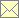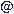Laser Doppler Data Processing Techniques - Weighting

 Home Scope Literature Data Programs Techniques Links Impressum Data Protection Policy Data Basewebmasternambisde 12.3.2018

In measurements with fluctuating velocity, e.g. in turbulent flows, the correlation between the instantaneous mean data rate and the convective velocity prefers values of high velocity and introduces a bias to all statistical quantities and functions derived from laser Doppler data. This bias can be reduced significantly by an appropriate weighting of the velocity samples. Examples of weighted averages are that of the mean velocity

$u ‾ = ∑ i w i u i ∑ i w i$

and that of the variance

$s 2 = ∑ i w i ( u i - u ‾ ) 2 ∑ i w i$

with the measured velocity samples $u i$ and their individual weights $w i$. Similar methods for implementing appropriate weighting factors exist also for correlation and spectral estimates.

Ideally, the weights invert the velocity-dependent probability of the measurement of a particular velocity sample. Different methods have been developed to estimate the instantaneous probability of the occurrence of a sample directly from quantities measured with the laser Doppler data set. Known methods are

where only Transit-Time Weighting has found broad acceptance.

review paper: